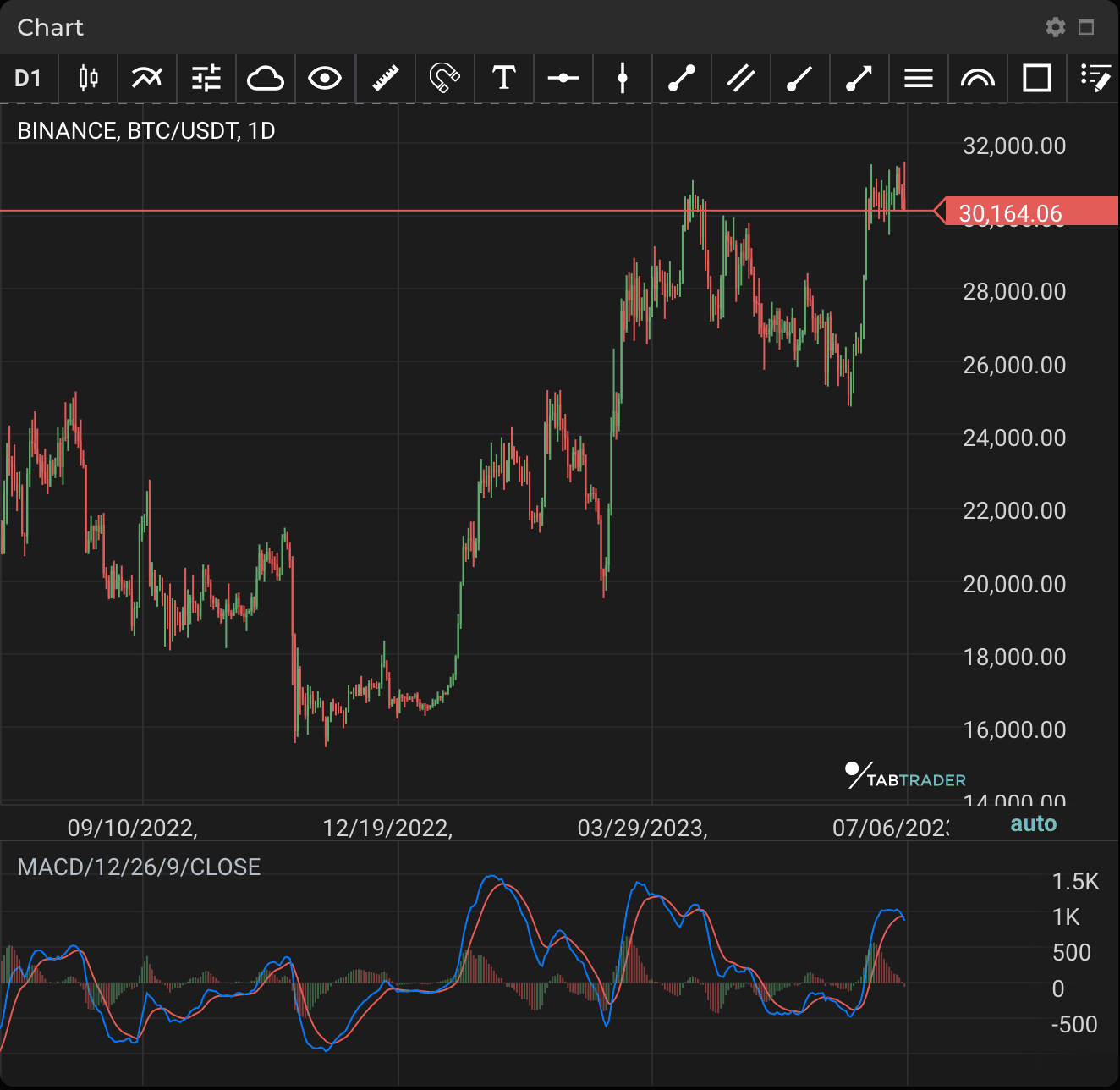# Moving Average Convergence Divergence

Publication date is

Moving Average Convergence/ Divergence (MACD) is a trend-based momentum indicator that shows the relationship between two moving averages of an asset’s price. MACD is calculated by subtracting the 26-period exponential moving average (EMA) from the 12-period EMA.

The result of that calculation is the MACD line. A 9-day EMA of the MACD called the "signal line” is then plotted on top of the MACD line, which can function as a trigger for buy and sell signals. Traders might choose to buy the asset when MACD crosses above its signal line and sell or short it when MACD crosses below the signal line.MACD

### Calculation

Moving Average Convergence/ Divergence=12-Period EMA − 26-Period EMA

MACD is calculated by means of the subtraction of two EMAs of different periods: the long-term EMA (by default 26 periods) from the short-term EMA (by default 12 periods).

Looking to get started in crypto trading?

Try TabTrader on mobile or web!